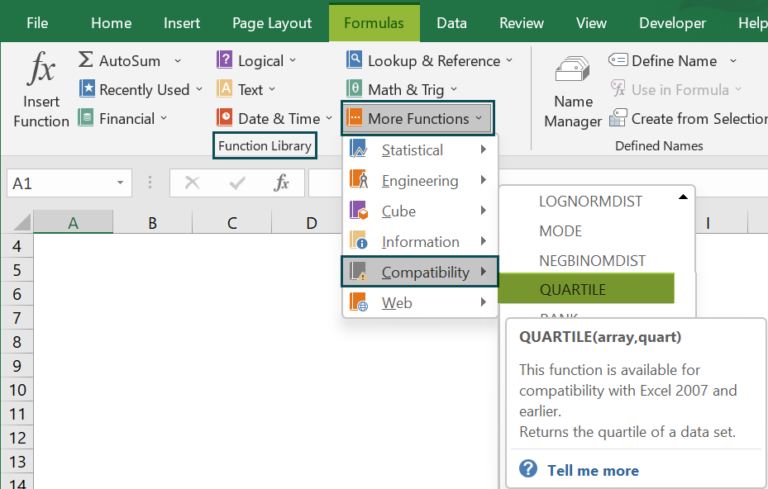# QUARTILE Excel FunctionArticle byExcelMojo Team## What Is QUARTILE Function In Excel?

The QUARTILE Function in Excel helps users find quartiles of a dataset. The different quartiles are the minimum value, first quartile [middle number between the minimum and median dataset], second quartile [median of the dataset], third quartile [middle value between the median of the dataset and the maximum], and maximum value.

The QUARTILE Excel function is a built-in function, so we can insert the formula from the “Function Library” or enter it directly in the worksheet. For example, we will apply the QUARTILE function to evaluate the equal portions.

Enter the formulas =QUARTILE(\$A\$2:\$A\$5,0) in cell B2, =QUARTILE(\$A\$2:\$A\$5,1) in cell B3, =QUARTILE(\$A\$2:\$A\$5,2) in cell B4, =QUARTILE(\$A\$2:\$A\$5,3) in cell B5, and =QUARTILE(\$A\$2:\$A\$5,4) in cell B6. Press “Enter” every time a formula is entered.

The output is shown above. Column C is for our reference.

###### Key Takeaways
• The QUARTILE Excel function is an inbuilt function available as a statistical function in Excel 2007 and before versions.
• The QUARTILE() is replaced with QUARTILE.EXC and QUARTILE.INC and is now available as a compatibility function.
• The function divides the dataset into four equal portions, namely, the minimum value, first quartile [middle number between the minimum and median dataset], second quartile [median of the dataset], third quartile [middle value between the median of the dataset and the maximum], and maximum value.
• The QUARTILE function returns a numeric value.

### QUARTILE() Excel Formula

The syntax of the QUARTILE Excel formula is,

The arguments of the QUARTILE Excel formula are,

• array: It is a mandatory argument. It is the dataset of numeric values to find the quartile.
• quart: It is a mandatory argument. It indicates the quartile value to be returned. It is an integer between 0 and 4, representing the required quartile.

### How to Use the QUARTILE Excel Function?

We can use the QUARTILE Excel Function in 2 methods, namely,

1. Access from the Excel ribbon.
2. Enter in the worksheet directly.

#### Method #1 – Access from the Excel ribbon

First, choose an empty cell → select the “Formulas” tab → go to the “Function Library” group → click the “More Functions” option drop-down → click the “Compatibility” option right arrow → select the “QUARTILE” function, as shown below.

The “Function Arguments” window appears. Enter the argument values in the “Array” and “Quart” fields → click “OK”, as shown below.

#### Method #2 – Enter in the worksheet manually

1. Select an empty cell for the output.
2. Type =QUARTILE( in the selected cell. [Alternatively, type =Q and double-click the QUARTILE function from the list of suggestions shown by Excel.
3. Enter the arguments as cell values or Excel cell references.
4. Close the brackets and press the “Enter” key.

Let us take an example to understand this function.

We will calculate Mr. Raj Mohan’s company sales data using QUARTILE Excel function.

In the table, the data is,

• Column A contains Sales.
• Column B contains Quartile Value.

The steps to calculate the equal portion using QUARTILE Excel function are as follows:

1. Select cell C2, enter the formula =QUARTILE(\$A\$2:\$A\$6,B2), [“\$A\$2:\$A\$6” is the array value.]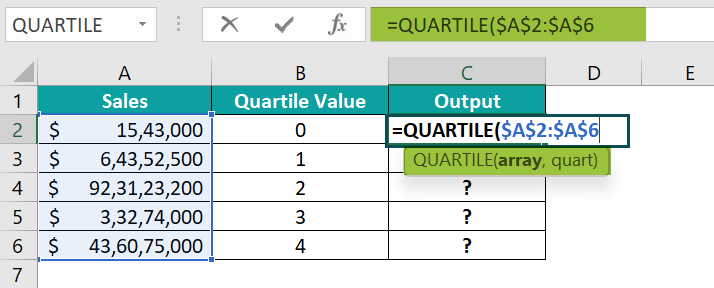2. In continuation with the formula, enter the value of ‘quart’ as “B2”, and close the brackets.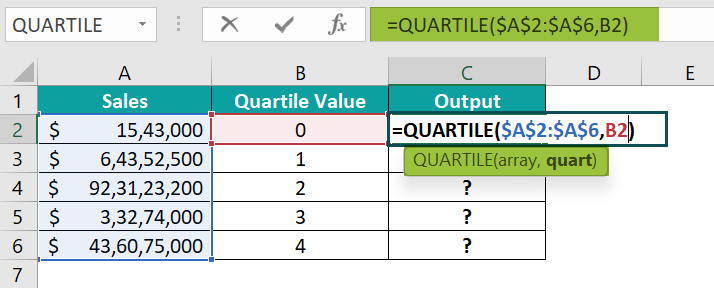3. Press the “Enter” key. The result is “1543000”, as shown below.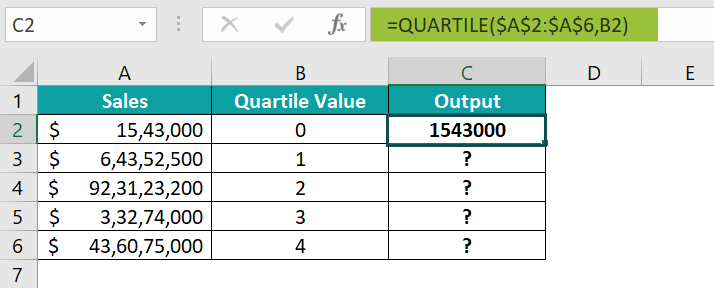4. Drag the formula from cell C2 to C6 using the fill handle.

[Note: If we enter the quart value from 0 to 4 directly in the formula, then we cannot copy the formula. However, if the quart values are entered in a column, like column B in the above table, we can enter as cell references and then drag the formula.]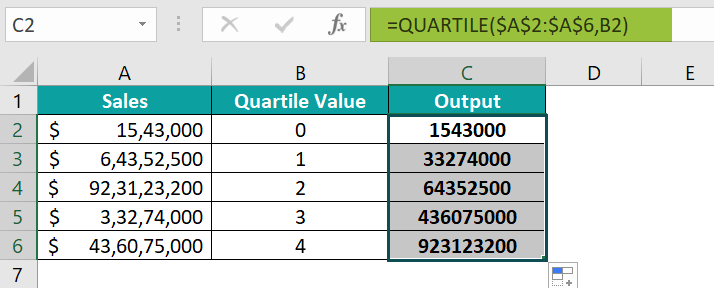The output is shown above.

### Examples

We will understand some advanced scenarios with QUARTILE Excel function examples.

#### Example #1

We will calculate the set data values, as the QUARTILE, as equal portions using the QUARTILE function.

In the table, the data is,

• Column A contains Value.
• Column B contains Quartile Value.

The steps to calculate the equal portion using the QUARTILE Excel function are as follows:

• Step 1:  Select cell C2, enter the formula =QUARTILE(\$A\$2:\$A\$10,B2), and press the “Enter” key. The result is ‘1’, as shown below.
• Step 2: Drag the formula from cell C2 to C6 using the fill handle.

The output is shown above.

#### Example #2

We will calculate the dates, as QUARTILE, as equal portions using the QUARTILE Excel function.

In the table, the data is,

• Column A contains the Value.
• Column B contains Quartile Value.

The steps to calculate the equal portion using the QUARTILE Excel function are as follows:

• Step 1:  Select cell C2, enter the formula =QUARTILE(\$A\$2:\$A\$6,B2), and press the “Enter” key. The result is “11-031980”, as shown below.
• Step 2: Drag the formula from cell C2 to C6 using the fill handle.

The output is shown above. We can format the output cells to “Date” to get accurate results.

#### Example #3

We will calculate the value as percentage data, as QUARTILE, as equal portions using the QUARTILE Excel function.

In the table, the data is,

• Column A contains Value.
• Column B contains Quartile Value.

The steps to calculate the equal portion using the QUARTILE function are as follows:

• Step 1:  Select cell C2, enter the formula =QUARTILE(\$A\$2:\$A\$6,B2), and press the “Enter” key. The result is “4%”, as shown below.
• Step 2: Drag the formula from cell C2 to C6 using the fill handle.

The output is shown above.

#### Example #4

We will calculate the value as time, as the QUARTILE, as equal portions using the QUARTILE Excel function.

In the table, the data is,

• Column A contains Value.
• Column B contains Quartile Value.

The steps to calculate the equal portion using the QUARTILE Excel function are as follows:

• Step 1:  Select cell C2, enter the formula =QUARTILE(\$A\$2:\$A\$6,B2), and press the “Enter” key. The result is shown below.
• Step 2: Drag the formula from cell C2 to C6 using the fill handle.

The output is shown below. We can format the output cells to “Time” to get accurate results.

### Important Things to Note

• We get the #VALUE! error occurs when the second argument value is non-numeric.
• The #NUM! error occurs,
• If the quart argument is greater than four or less than 0.
• If the given array is empty.
• In multiple values datasets, if we enter the quart value from 0 to 4 directly in the formula, we cannot copy or drag the formula, as we will get incorrect output. Therefore, create a column with the required quart values, and in the formula, enter the cell reference, and then drag the formula.

1. What does the QUARTILE function do in Excel?

The QUARTILE Excel function returns the quartile of a given data set. The financial analyst uses the function to determine a specific percentage of income in a population. The function is very useful in revenue analysis.

The syntax of the QUARTILE function is =QUARTILE(array,quart)

2. How does the QUARTILE function work in Excel?

We can use the QUARTILE Excel function as follows:
1. Select an empty cell for the output.
2. Type =QUARTILE( in the selected cell. [Alternatively, type =Q and double-click the QUARTILE function from the list of suggestions shown by Excel.
3. Enter the arguments as cell values or cell references.
4. Close the brackets and press the “Enter” key.

For example, we will evaluate the equal portions of the given values in the below table using the QUARTILE Excel function.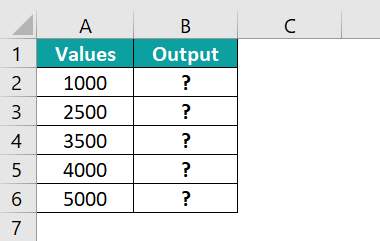Enter the formulas, =QUARTILE(\$A\$2:\$A\$6,0) in cell B2,  =QUARTILE(\$A\$2:\$A\$6,1) in cell B3, =QUARTILE(\$A\$2:\$A\$6,2) in cell B4, =QUARTILE(\$A\$2:\$A\$6,3) in cell B4, and =QUARTILE(\$A\$2:\$A\$6,4) in cell B5. Press the “Enter” key every time a formula is entered.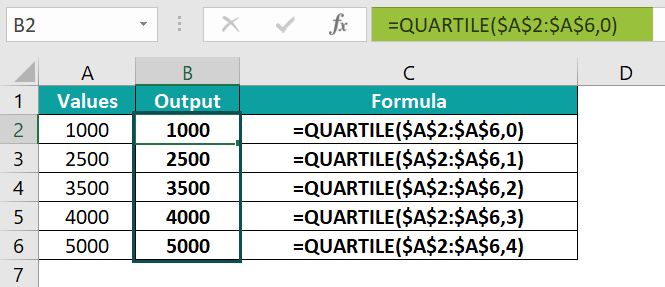The output is shown above. Column C is for our reference.

3. Where is the QUARTILE Function in Excel?

The QUARTILE Function in Excel is found in the formulas tab, as follows:

First, choose an empty cell → select the “Formulas” tab → go to the “Function Library” group → click the “More Functions” option drop-down → click the “Compatibility” option right arrow à select the “QUARTILE” function, as shown below.